Courses

# Patterns - 1

## 20 Questions MCQ Test Science Olympiad Class 4 | Patterns - 1

Description
This mock test of Patterns - 1 for Class 4 helps you for every Class 4 entrance exam. This contains 20 Multiple Choice Questions for Class 4 Patterns - 1 (mcq) to study with solutions a complete question bank. The solved questions answers in this Patterns - 1 quiz give you a good mix of easy questions and tough questions. Class 4 students definitely take this Patterns - 1 exercise for a better result in the exam. You can find other Patterns - 1 extra questions, long questions & short questions for Class 4 on EduRev as well by searching above.
QUESTION: 1

### Find the odd one out.

Solution:

D+1 = E, G + 1 = H, L + 1 = M but O – 1 = N.

QUESTION: 2

### Find the odd one out.

Solution: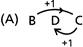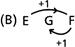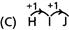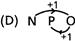Hence C is odd one out.

QUESTION: 3

### Find the odd one out.

Solution:

R – 1 = Q, N – 1 = M, J – 1 = I but P + 1 = Q.

QUESTION: 4

Find the odd one out.

Solution:

V + 1 = W, S + 1 = T, P + 1= Q but X+2 = Z.

QUESTION: 5

Find the odd one out.

Solution:

Pattern is reverse writing of letters. Z←Y←X; W←V←U; T←S←R but it is not so in case of LMC.

QUESTION: 6

Find the odd one out.

Solution:

Pattern is writing continuous three digits. 2→3→4; 3→4→5; 4→5→6 but it is not so in came of D.

QUESTION: 7

Find the odd one out.

Solution:
QUESTION: 8

Find the odd one out.

Solution:
QUESTION: 9

Find the odd one out.

Solution:
QUESTION: 10

Find the odd one out.

Solution:

Except for option (D), rest are all are palindrome numbers. Palindrome are the numbers which are same when read from either side.  Example: 12421.

QUESTION: 11

Find the odd one out.

Solution:

Roof is not a part of the plant.

QUESTION: 12

Find the odd one out.

Solution:

Maths is a subject while other options are stationary items.

QUESTION: 13

Find the odd one out.

Solution:

Cycle has only two wheels and moves on pedaling.

QUESTION: 14

Find the odd one out.

Solution:

Walk is done by foot unlike other options which are done by transport.

QUESTION: 15

Find the odd one out.

Solution:

All except Bridge are used for up and down movement.

QUESTION: 16

Find the letter which will end the first word and start the second word.
BE?AS

Solution:

The answer is G as it completes the first word; BEG and also begins the second word GAS.

QUESTION: 17

Find the letter which will end the first word and start the second word.
BOT?URT

Solution:

The answer is H as it completes the first word; BOTH and also begins the second word HURT.

QUESTION: 18

Find the letter which will end the first word and start the second word.
​SHAR?RINT

Solution:

The answer is P as it completes the first word; SHARP and also begins the second word PRINT.

QUESTION: 19

Find the letter which will end the first word and start the second word.
​CAME?IGHT

Solution:

The answer is L as it completes the first word; CAMEL and also begins the second word LIGHT.

QUESTION: 20

Find the letter which will end the first word and start the second word.
HAN?ATE

Solution:

The answer is D as it completes the first word; HAND and also begins the second word DATE.

Track your progress, build streaks, highlight & save important lessons and more!

### Similar Content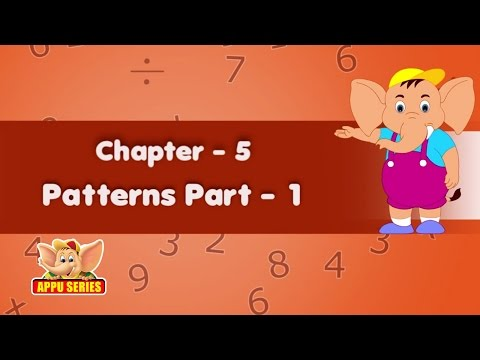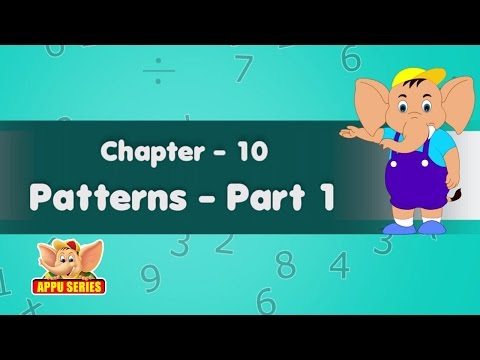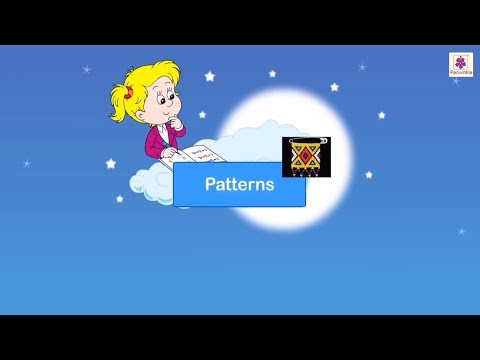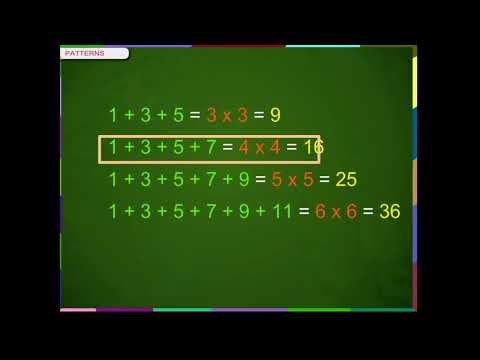### Related tests